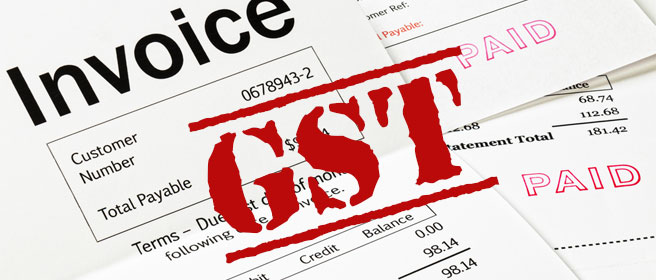# What is HSN code under GST?The full form of HSN code means Harmonized System Nomenclature.

This post explains about HSN codes classification into 2 digit HSN code, 4 digit HSN codes, 6 digit HSN codes and 8 digit HSN Code number.

HSN code number is widely used in many countries to classify goods for taxation purpose, claiming benefits etc. Harmonized System of Nomenclature number for each commodity is accepted by most of the countries and the said HSN number remains same for almost all goods. However, HSN number used in some of the countries varies little, based on the nature of goods classified.

In India, when implementing GST, all goods are classified based on HSN number for GST purpose. At present, HSN number is mainly used for classifying goods to compute Value Added Tax in India. The same classification code (HSN) is adopted for classifying Goods and Service Tax, GST.

When preparing Tax Invoice for GST, the HSN number must be mentioned on GST invoice.

HSN Code, How many digits? 2 digit HSN code, 4 Digit HSN code, 6 digit HSN code or 8 digit HSN code?

About 21 groups of HSN codes are classified. These 21 sections of HSN codes are sub classified in to 99 Chapters of HSN code. Each Chapter can be named as two digit HSN number. Again each two digit HSN number is sub classified to 4 digit HSN code. Based on such 4 digit HSN codes, the sub classification is done as 6 digit HSN code.

As per Harmonized System of Nomenclature accepted world wide, normally 6 digit HSN code is accepted worldwide. However, in most of the countries additional two digit HSN code is added at last part of such HSN code number to sub classify 6 digit HSN code number.

HSN numbers are mainly used for taxation purpose especially for Import and Export worldwide. As explained, 6 digit HSN code is accepted for Export and Import calculation of duties and levies worldwide. However in India and some of the countries, 8 digit HSN codes are used for computation of Tax for Import and Export.

The above information describes about HSN numbers and its classification as 2 digit HSN code, 4 digit HSN codes, 6 digit HSN codes and 8 digit HSN codes.